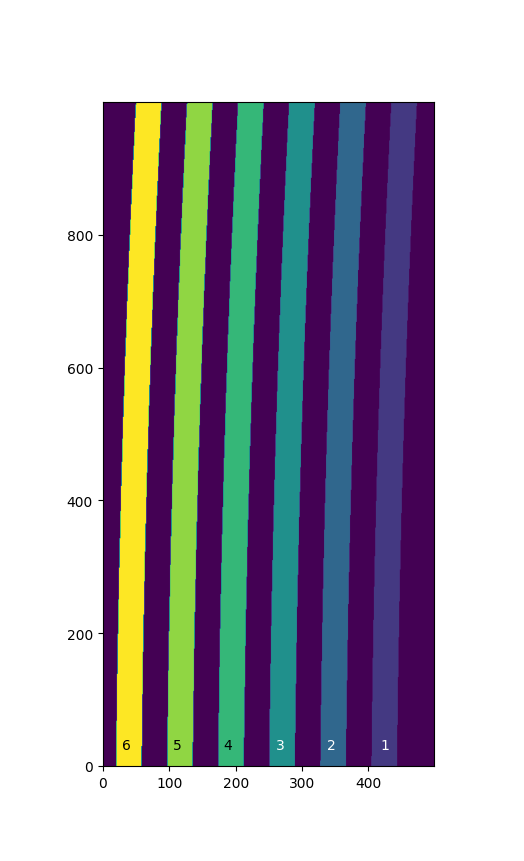# An IFU Example - managing a discontiguous WCS¶

An IFU image represents the projection of several slices on a detector. Between the slices there are pixels which don’t belong to any slice. In general each slice has a unique WCS transform. There are two ways to represent this kind of transforms in GWCS depending on the way the instrument is calibrated and the available information.

## Using a pixel to slice mapping¶

In this case a pixel map associating each pixel with a slice label (number or string) is available. The image below represents the projection of the slits of an IFU on a detector with a size (500, 1000). Slices are labeled from 1 to 6, while label 0 is reserved for pixels between the slices.There are several models in GWCS which are useful in creating a WCS.

Given (x, y) pixel indices, `LabelMapperArray` returns labels (int or str) associated with these indices. `RegionsSelector` maps labels with transforms. It uses the `LabelMapperArray` to map these transforms to pixel indices.

A step by step example of constructing the WCS for an IFU with 6 slits follows.

First, import the usual packages.

```>>> import numpy as np
>>> from astropy.modeling import models
>>> from astropy import coordinates as coord
>>> from astropy import units as u
>>> from gwcs import wcs, selector
>>> from gwcs import coordinate_frames as cf
```

The output frame is common for all slits and is a composite frame with two subframes, `CelestialFrame` and `SpectralFrame`.

```>>> sky_frame = cf.CelestialFrame(name='icrs', reference_frame=coord.ICRS(), axes_order=(0, 2))
>>> spec_frame = cf.SpectralFrame(name='wave', unit=(u.micron,), axes_order=(1,), axes_names=('lambda',))
>>> cframe = cf.CompositeFrame([sky_frame, spec_frame], name='world')
>>> det = cf.Frame2D(name='detector')
```

All slices have the same input and output frames, however each slices has a different model transforming from pixels to world coordinates (RA, lambda, dec). For the sake of brevity this example uses a simple shift transform for each slice. Detailed examples of how to create more realistic transforms are available in Adding distortion to the imaging example.

```>>> transforms = {}
>>> for i in range(1, 7):
...     transforms[i] = models.Mapping([0, 0, 1]) | models.Shift(i * 0.1) & models.Shift(i * 0.2) & models.Scale(i * 0.1)
```

One way to initialize `LabelMapperArray` is to pass it the shape of the array and the vertices of each slit on the detector {label: vertices} see :meth: `from_vertices`. In this example the mask is an array with the size of the detector where each item in the array corresponds to a pixel on the detector and its value is the slice number (label) this pixel belongs to.

Assuming the array is stored in ASDF format, create the mask:

Create the pixel to world transform for the entire IFU:

```>>> regions_transform = selector.RegionsSelector(inputs=['x','y'],
...                                              outputs=['ra', 'dec', 'lam'],
...                                              selector=transforms,
...                                              undefined_transform_value=np.nan)
```

The WCS object now can evaluate simultaneously the transforms of all slices.

```>>> wifu = wcs.WCS(forward_transform=regions_transform, output_frame=cframe, input_frame=det)
>>> y, x = np.mgrid[:y, :x]
>>> r, d, l = wifu(x, y)
```

or of single slices.

The `set_input()` method returns the forward_transform for a specific label.

```>>> wifu.forward_transform.set_input(4)(1, 2)
(1.4, 1.8, 0.8)
```

## Custom model storing transforms in a dictionary¶

In case a pixel to slice mapping is not available, one can write a custom mdoel storing transforms in a dictionary. The model would look like this:

```from astropy.modeling.core import Model
from astropy.modeling.parameters import Parameter

class CustomModel(Model):

inputs = ('label', 'x', 'y')
outputs = ('xout', 'yout')

def __init__(self, labels, transforms):
super().__init__()
self.labels = labels
self.models = models

def evaluate(self, label, x, y):
index = self.labels.index(label)
return self.models[index](x, y)
```